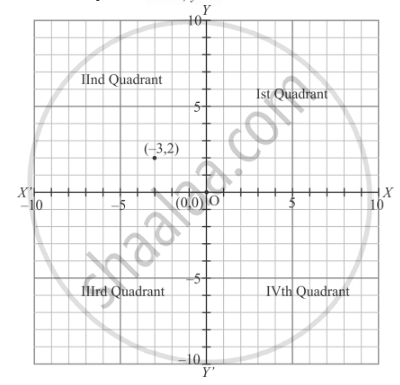# A Point Whose Abscissa is −3 and Ordinate 2 Lies in - Mathematics

MCQ

A point whose abscissa is −3 and ordinate 2 lies in

#### Solution

As we know that

In the first quadrant   x > 0, y >0

In the second quadrant  x < 0 , y > 0

In the third quadrant  x < 0 y < 0

In the fourth quadrant  x > 0,y < 0The point whose abscissa is −3 which is negative and ordinate 2 is positive, so this point lies in the second quadrant.

Concept: Coordinate Geometry
Is there an error in this question or solution?

#### APPEARS IN

RD Sharma Mathematics for Class 9
Chapter 8 Co-ordinate Geometry
Exercise 8.2 | Q 9 | Page 7

Share